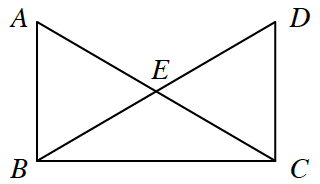### Home > INT2 > Chapter 8 > Lesson 8.1.1 > Problem8-8

8-8.

In the figure below, $\overline{AB} ≅ \overline{DC}$ and $∠ABC ≅ ∠DCB$. 8-8 HW eTool (Desmos). Homework Help ✎1. Is $\overline{AC} ≅ \overline{DB}$ ? Prove your answer using a flowchart or two-column proof.

Mark the given information on the diagram. Remember that $\overline{BC} ≅ \overline{CB}$ because of the Reflexive Property.

1. Do the measures of $∠ABC$ and $∠DCB$ make any difference in your solution to part (a)? Explain why or why not.

Explore using the eTool below.
Click the link to the right for full version. 8-8 HW eTool